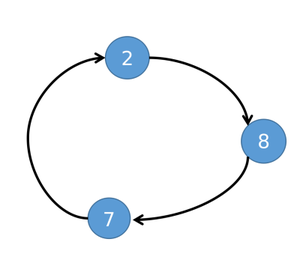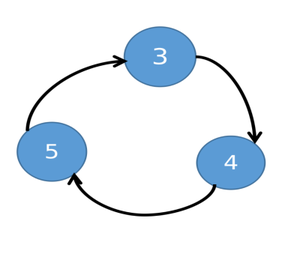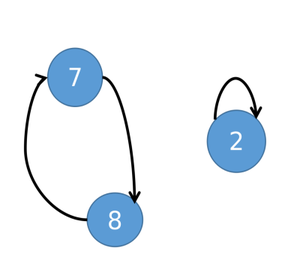# Minimize cost to sort an Array by swapping any pair of element (X, Y) with cost as (X + Y)

• Difficulty Level : Expert
• Last Updated : 20 Aug, 2021

Given an array arr[] consisting of N integers, the task is to find the minimum cost to sort the given array arr[] in ascending order by swapping any pair of elements (X, Y) such that the cost of swapping is (X + Y).

Examples:

Attention reader! Don’t stop learning now. Get hold of all the important DSA concepts with the DSA Self Paced Course at a student-friendly price and become industry ready.  To complete your preparation from learning a language to DS Algo and many more,  please refer Complete Interview Preparation Course.

In case you wish to attend live classes with experts, please refer DSA Live Classes for Working Professionals and Competitive Programming Live for Students.

Input: arr[] = {3, 2, 1}
Output: 4
Explanation:
Following are the swapping of array elements performed to sort the array:

1. Swapping the array elements at index 0 and 2 modifies the array to {1, 2, 3}. The cost of this swapping operation is (arr + arr) = (3 + 1) = 4.

After the above steps, the given array is sorted and the total cost is 4, which is the minimum among all possible combinations of swapping.

Input: arr[] = {7, 9, 15}
Output: 0

Approach:  The given problem can be solved based on the following observations:

1. Form a directed graph by forming edges between every ith element of the current array and the sorted array.
2. It can be observed that every component will always form a cycle as there every node will have in-degree and out-degree equal to 1.
3. Therefore, the idea is to sort the elements of each cycle separately.

Illustration:

• Suppose the given array is {8, 4, 5, 3, 2, 7}. The sorted array will be equal to the {2, 3, 4, 5, 7, 8}.
• For the above array, the graph will contain two components which are cycles.• If two elements are swapped in a cycle, of length K > 1 such that at least 1 element of this cycle will go to its destination. Then after the swap, it will be split into two cycles, one with length K – 1 and another one with length 1.
• For the given array, If 2 and 8 are swapped of 2 → 8 → 7 → 2 cycles. 2 will go to its destination, and 7 → 8 → 7 will form a smaller cycle.• Therefore, the minimum number of swaps needed to sort a cycle of size K is equal to the (K-1).
• It can be observed that each swap will add two elements to the cost. So 2 × (K – 1) elements will be added to the cost and there are K elements. So some elements will be added multiple times to the cost.
• Therefore, the idea is to, swap with the minimum value of a cycle with every other element to place them at the correct positions. Then in the final cost, every element will be added once and the minimum element will be added K – 1 time. So this is the optimal approach to solve a cycle.
• For sorting a cycle there are two choices: either to use only the local minimum of the cycle or to use both local and overall minimum of the array.

Follow the steps below to solve the problem:

• Initialize a variable res as 0 to store the total cost.
• Copy every element of arr[] to another array, say copyArr[] and sort copyArr[] in ascending order.
• Initialize a hashmap say place and store array elements and the correct position of it in the sorted array as key-value pair.
• Also, Initialize an array, visited[] of size N, and mark all its entries as false.
• Iterate over the array, arr[] using the variable i, and perform the following steps:
• If visited[i] is true then continue.
• If the element visited index is visited true and perform the following steps:
• If place[arr[i]] is equal to i then mark visited[i], true and continue.
• Initialize a variable say min_value as arr[i] and sum as 0, to store the minimum value of the current cycle and the sum of the elements of the cycle.
• Also, initialize a variable j as i to iterate over the cycle and a variable num as 0 to store the count of numbers in the cycle.
• Iterate until visited[j] is not true and in each iteration perform the following steps:
• Increment sum by arr[j] and num by 1 and then mark the visited[j] as true.
• Update min_value to min(min_value, arr[j]). And then assign value of place[arr[j]] to j.
• Decrement sum by the min_value.
• Now find the cost obtained by using the local minimum, min_value, and store it in a variable say Cost1.
• Cost1 = sum + min_val*(num-1).
• Now find the cost obtained by using the global minimum, copyArr, and store it in a variable say Cost2.
• Cost2 = copyArr * (num + 1) + 2 * min_val+ sum
• Now increment res by min(Cost1, Cost2).
• After completing the above steps, print the res as the total cost.

Below is the implementation of the above approach:

## C++

 `// C++ program for the above approach`   `#include ` `using` `namespace` `std;`   `// Function to find the minimum cost to` `// sort the array` `int` `findMinimumCost(``int` `arr[], ``int` `N)` `{` `    ``// Stores the required result` `    ``int` `res = 0;`   `    ``// Create 2 arrays` `    ``int` `copyArr[N], visited[N];`   `    ``for` `(``int` `i = 0; i < N; ++i) {` `        ``copyArr[i] = arr[i];` `        ``visited[i] = ``false``;` `    ``}`   `    ``// Sort the array, copyArr[] in` `    ``// increasing order` `    ``sort(copyArr, copyArr + N);`   `    ``// Map the numbers to their desired` `    ``// place after sorting` `    ``map<``int``, ``int``> place;`   `    ``// Store the original places of the` `    ``// elements in map` `    ``for` `(``int` `i = 0; i < N; ++i) {` `        ``place[copyArr[i]] = i;` `    ``}`   `    ``// Iterate in the range [0, N-1]` `    ``for` `(``int` `i = 0; i < N; ++i) {`   `        ``// If the ith index is not visited` `        ``if` `(visited[i] == ``false``) {`   `            ``// If the original place and` `            ``// the place in sorted array` `            ``// is same then only mark this` `            ``// element as visited` `            ``if` `(place[arr[i]] == i) {` `                ``visited[i] = ``true``;` `                ``continue``;` `            ``}`   `            ``// Else a new cycle is present` `            ``int` `min_val = arr[i], cost1, cost2;` `            ``int` `num = 0;` `            ``int` `sum = 0;` `            ``int` `j = i;`   `            ``// Iterate while the nodes` `            ``// in the current cycle is` `            ``// not visited` `            ``while` `(visited[j] == ``false``) {`   `                ``// Increment sum by arr[j]` `                ``sum += arr[j];` `                ``num++;`   `                ``// Update the min_val value` `                ``if` `(arr[j] < min_val) {` `                    ``min_val = arr[j];` `                ``}`   `                ``// Mark j as visited` `                ``visited[j] = ``true``;`   `                ``// Place j at its` `                ``// original place` `                ``j = place[arr[j]];` `            ``}`   `            ``// Sum of all numbers of` `            ``// cycle other than minimum` `            ``sum -= min_val;`   `            ``// Cost from local minimum` `            ``cost1 = sum + min_val * (num - 1);`   `            ``// Cost from overall minimum` `            ``cost2 = copyArr * (num + 1) + 2 * min_val` `                    ``+ sum;`   `            ``// Add the lower cost to` `            ``// the final result` `            ``if` `(cost1 < cost2) {` `                ``res += cost1;` `            ``}` `            ``else` `{` `                ``res += cost2;` `            ``}` `        ``}` `    ``}`   `    ``// Print the minimum cost` `    ``return` `res;` `}`   `// Driver Code` `int` `main()` `{` `    ``int` `arr[] = { 3, 2, 1 };` `    ``int` `N = (``sizeof``(arr) / ``sizeof``(``int``));` `    ``cout << findMinimumCost(arr, N);`   `    ``return` `0;` `}`

## Java

 `import` `java.util.ArrayList;` `import` `java.util.Arrays;` `import` `java.util.HashMap;` `import` `java.util.Map;`   `//Java program for above approach` `class` `GFG{`   `    ``// Function to find the minimum cost to` `// sort the array` `    ``static` `int` `findMinimumCost(``int``[] arr, ``int` `N)` `    ``{` `        ``// Stores the required result` `        ``int` `res = ``0``;`   `        ``// Create 2 arrays` `        ``int``[] copyArr = ``new` `int``[N];` `        ``boolean``[] visited = ``new` `boolean``[N];`   `        ``for` `(``int` `i = ``0``; i < N; ++i) {` `            ``copyArr[i] = arr[i];` `            ``visited[i] = ``false``;` `        ``}`   `        ``// Sort the array, copyArr[] in` `        ``// increasing order` `        ``Arrays.sort(copyArr);`   `        ``// Map the numbers to their desired` `        ``// place after sorting` `        ``Map place = ``new` `HashMap<>();`   `        ``// Store the original places of the` `        ``// elements in map` `        ``for` `(``int` `i = ``0``; i < N; ++i) {` `            ``place.put(copyArr[i],i);` `        ``}`   `        ``// Iterate in the range [0, N-1]` `        ``for` `(``int` `i = ``0``; i < N; ++i) {`   `            ``// If the ith index is not visited` `            ``if` `(visited[i] == ``false``) {`   `                ``// If the original place and` `                ``// the place in sorted array` `                ``// is same then only mark this` `                ``// element as visited` `                ``if` `(place.get(arr[i]) == i) {` `                    ``visited[i] = ``true``;` `                    ``continue``;` `                ``}`   `                ``// Else a new cycle is present` `                ``int` `min_val = arr[i], cost1, cost2;` `                ``int` `num = ``0``;` `                ``int` `sum = ``0``;` `                ``int` `j = i;`   `                ``// Iterate while the nodes` `                ``// in the current cycle is` `                ``// not visited` `                ``while` `(visited[j] == ``false``) {`   `                    ``// Increment sum by arr[j]` `                    ``sum += arr[j];` `                    ``num++;`   `                    ``// Update the min_val value` `                    ``if` `(arr[j] < min_val) {` `                        ``min_val = arr[j];` `                    ``}`   `                    ``// Mark j as visited` `                    ``visited[j] = ``true``;`   `                    ``// Place j at its` `                    ``// original place` `                    ``j = place.get(arr[j]);` `                ``}`   `                ``// Sum of all numbers of` `                ``// cycle other than minimum` `                ``sum -= min_val;`   `                ``// Cost from local minimum` `                ``cost1 = sum + min_val * (num - ``1``);`   `                ``// Cost from overall minimum` `                ``cost2 = copyArr[``0``] * (num + ``1``) + ``2` `* min_val` `                        ``+ sum;`   `                ``// Add the lower cost to` `                ``// the final result` `                ``if` `(cost1 < cost2) {` `                    ``res += cost1;` `                ``}` `                ``else` `{` `                    ``res += cost2;` `                ``}` `            ``}` `        ``}`   `        ``// Print the minimum cost` `        ``return` `res;` `    ``}`   `    ``// Driver Code` `    ``public` `static` `void` `main(String[] args) {` `        ``int``[] arr = { ``3``, ``2``, ``1` `};` `        ``int` `N = arr.length;` `        ``System.out.println(findMinimumCost(arr, N));`   `    ``}` `}`   `// This code is contributed by hritikrommie.`

## Python3

 `# Python program for the above approach`   `# Function to find the minimum cost to` `# sort the array` `def` `findMinimumCost(arr, N):` `    ``# Stores the required result` `    ``res ``=` `0`   `    ``# Create 2 arrays` `    ``copyArr ``=` `[``0``] ``*` `N` `    ``visited ``=` `[``0``] ``*` `N`   `    ``for` `i ``in` `range``(N):` `        ``copyArr[i] ``=` `arr[i]` `        ``visited[i] ``=` `False`   `    ``# Sort the array, copyArr[] in` `    ``# increasing order` `    ``copyArr.sort()`   `    ``# Map the numbers to their desired` `    ``# place after sorting` `    ``place ``=` `{}`   `    ``# Store the original places of the` `    ``# elements in map` `    ``for` `i ``in` `range``(N):` `        ``place[copyArr[i]] ``=` `i`   `    ``# Iterate in the range [0, N-1]` `    ``for` `i ``in` `range``(N):`   `        ``# If the ith index is not visited` `        ``if` `(visited[i] ``=``=` `False``):`   `            ``# If the original place and` `            ``# the place in sorted array` `            ``# is same then only mark this` `            ``# element as visited` `            ``if` `(place[arr[i]] ``=``=` `i):` `                ``visited[i] ``=` `True` `                ``continue`   `            ``# Else a new cycle is present` `            ``min_val ``=` `arr[i]` `            ``num ``=` `0` `            ``sum` `=` `0` `            ``j ``=` `i`   `            ``# Iterate while the nodes` `            ``# in the current cycle is` `            ``# not visited` `            ``while` `(visited[j] ``=``=` `False``):`   `                ``# Increment sum by arr[j]` `                ``sum` `+``=` `arr[j]` `                ``num ``+``=` `1`   `                ``# Update the min_val value` `                ``if` `(arr[j] < min_val):` `                    ``min_val ``=` `arr[j]`   `                ``# Mark j as visited` `                ``visited[j] ``=` `True`   `                ``# Place j at its` `                ``# original place` `                ``j ``=` `place[arr[j]]`   `            ``# Sum of all numbers of` `            ``# cycle other than minimum` `            ``sum` `-``=` `min_val`   `            ``# Cost from local minimum` `            ``cost1 ``=` `sum` `+` `min_val ``*` `(num ``-` `1``)`   `            ``# Cost from overall minimum` `            ``cost2 ``=` `copyArr[``0``] ``*` `(num ``+` `1``) ``+` `2` `*` `min_val ``+` `sum`   `            ``# Add the lower cost to` `            ``# the final result` `            ``if` `(cost1 < cost2):` `                ``res ``+``=` `cost1` `            ``else``:` `                ``res ``+``=` `cost2`   `    ``# Print the minimum cost` `    ``return` `res`     `# Driver Code`   `arr ``=` `[``3``, ``2``, ``1``]` `N ``=` `len``(arr)` `print``(findMinimumCost(arr, N))`     `# This code is contributed by gfgking`

## C#

 `// C# program for the above approach` `using` `System;` `using` `System.Collections.Generic;`   `class` `GFG{` `    `  `// Function to find the minimum cost to` `// sort the array` `static` `int` `findMinimumCost(``int``[] arr, ``int` `N)` `{` `    `  `    ``// Stores the required result` `    ``int` `res = 0;`   `    ``// Create 2 arrays` `    ``int``[] copyArr = ``new` `int``[N]; ` `    ``int``[] visited = ``new` `int``[N]; `   `    ``for``(``int` `i = 0; i < N; ++i) ` `    ``{` `        ``copyArr[i] = arr[i];` `        ``visited[i] = 0;` `    ``}`   `    ``// Sort the array, copyArr[] in` `    ``// increasing order` `    ``Array.Sort(copyArr);`   `    ``// Map the numbers to their desired` `    ``// place after sorting` `    ``Dictionary<``int``,` `               ``int``> place = ``new` `Dictionary<``int``,` `                                           ``int``>();`   `    ``// Store the original places of the` `    ``// elements in map` `    ``for``(``int` `i = 0; i < N; ++i)` `    ``{` `        ``place[copyArr[i]] = i;` `    ``}`   `    ``// Iterate in the range [0, N-1]` `    ``for``(``int` `i = 0; i < N; ++i)` `    ``{` `        `  `        ``// If the ith index is not visited` `        ``if` `(visited[i] == 0)` `        ``{` `            `  `            ``// If the original place and` `            ``// the place in sorted array` `            ``// is same then only mark this` `            ``// element as visited` `            ``if` `(place[arr[i]] == i) ` `            ``{` `                ``visited[i] = 1;` `                ``continue``;` `            ``}`   `            ``// Else a new cycle is present` `            ``int` `min_val = arr[i], cost1, cost2;` `            ``int` `num = 0;` `            ``int` `sum = 0;` `            ``int` `j = i;`   `            ``// Iterate while the nodes` `            ``// in the current cycle is` `            ``// not visited` `            ``while` `(visited[j] == 0)` `            ``{` `                `  `                ``// Increment sum by arr[j]` `                ``sum += arr[j];` `                ``num++;`   `                ``// Update the min_val value` `                ``if` `(arr[j] < min_val) ` `                ``{` `                    ``min_val = arr[j];` `                ``}`   `                ``// Mark j as visited` `                ``visited[j] = 1;`   `                ``// Place j at its` `                ``// original place` `                ``j = place[arr[j]];` `            ``}`   `            ``// Sum of all numbers of` `            ``// cycle other than minimum` `            ``sum -= min_val;`   `            ``// Cost from local minimum` `            ``cost1 = sum + min_val * (num - 1);`   `            ``// Cost from overall minimum` `            ``cost2 = copyArr * (num + 1) + ` `                             ``2 * min_val + sum;`   `            ``// Add the lower cost to` `            ``// the final result` `            ``if` `(cost1 < cost2) ` `            ``{` `                ``res += cost1;` `            ``}` `            ``else` `            ``{` `                ``res += cost2;` `            ``}` `        ``}` `    ``}`   `    ``// Print the minimum cost` `    ``return` `res;` `}` `    `  `// Driver code` `public` `static` `void` `Main()` `{` `    ``int``[] arr = { 3, 2, 1 };` `    ``int` `N = arr.Length;` `    `  `    ``Console.WriteLine(findMinimumCost(arr, N));` `}` `}`   `// This code is contributed by sanjoy_62`

## Javascript

 ``

Output:

`4`

Time Complexity: O(N*log N)
Auxiliary Space: O(N)

My Personal Notes arrow_drop_up
Recommended Articles
Page :